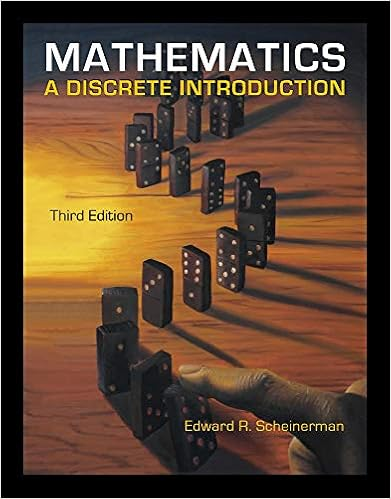# Approximate the three real eigenvalues of a to the

• Notes
• 26

This preview shows page 20 - 22 out of 26 pages.

##### We have textbook solutions for you!
The document you are viewing contains questions related to this textbook.The document you are viewing contains questions related to this textbook.
Chapter 9 / Exercise 49.14
Mathematics: A Discrete Introduction
ScheinermanExpert Verified
Approximate the three real eigenvalues of A to the nearest integer. One of the three eigenvalues of A is negative. Find a good approximation for this eigen value and a corresponding eigenvector by using the procedure outlined in part (a). You are not asked to do the same for the two other eigenvalues. 35. Demonstrate the formula tr A = k j -f- k.2 + • • • + k n, where the A/ are the complex eigenvalues of the matrix A, counted with their algebraic multiplicities. 1 2 3 4 5 6 7 8 10
##### We have textbook solutions for you!
The document you are viewing contains questions related to this textbook.The document you are viewing contains questions related to this textbook.
Chapter 9 / Exercise 49.14
Mathematics: A Discrete Introduction
ScheinermanExpert Verified
356 C H A PTER 7 Eigenvalues and Eigenvectors 36. Hint: Consider the coefficient of k n~ 1 in (A.) = (k\ — k)(k 2 A .) • • • (kn —k)y and compare the result with Fact 7.2.5. In 1990, the population of the African country Benin was about 4.6 million people. Its composition by age was as follows: Age Bracket 0-15 15-30 30-45 45-60 60-75 75-90 Percent of 46.6 25.714.78.43.80.8 Population We represent these data in a state vector whose compo nents are the populations in the various age brackets, in millions: i(0) = 4.6 0.466 0.257 0.147 0.084 0.038 0.008 2.14 1.18 0.68 0.39 0.17 0.04 1.1 1.6 0.6 0 0 0 0.82 0 0 0 0 0 0 0.89 0 0 0 0 0 0 0.81 0 0 0 0 0 0 0.53 0 0 0 0 0 0 0.29 0 We measure time in increments of 15 years, with t = 0 in 1990. For example, jc(3) gives the age composition in the year 2035 (1990 + 3 15). If current age-dependent birth and death rates are extrapolated, we have the fol lowing model: i(/ + l) = = Ax(t). a. Explain the significance of all the entries in the ma trix A in terms of population dynamics. b. Find the eigenvalue of A with largest modulus and an associated eigenvector. (Use technology.) What is the significance of these quantities in terms of popula tion dynamics? (For a summary on matrix techniques used in the study of age-structured populations, see Dmitrii O. Logofet, Matrices and Graphs: Stability Problems in Mathematical Ecology, Chapters 2 and 3, CRC Press, 1993.) 37. Consider the set IHI of all complex 2 x 2 matrices of the form A = - z w where w and z are arbitrary complex numbers. a. Show that IHI is closed under addition and multiplica tion. (That is, show that the sum and the product of two matrices in IHI are again in IHI.) b. Which matrices in H are invertible? C 4 = c. If a matrix in HI is invertible, is the inverse in IHI as well? d. Find two matrices A and B in M such that AB ^ BA. IHI is an example of a skew field: It satisfies all ax ioms for a field, except for the commutativity of mul tiplication. [The skew field IHI was introduced by the Irish mathematician Sir William Hamilton (1805- 1865); its elements are called the quaternions. An other way to define the quaternions is discussed in Exercise 5.3.64.]
•••